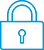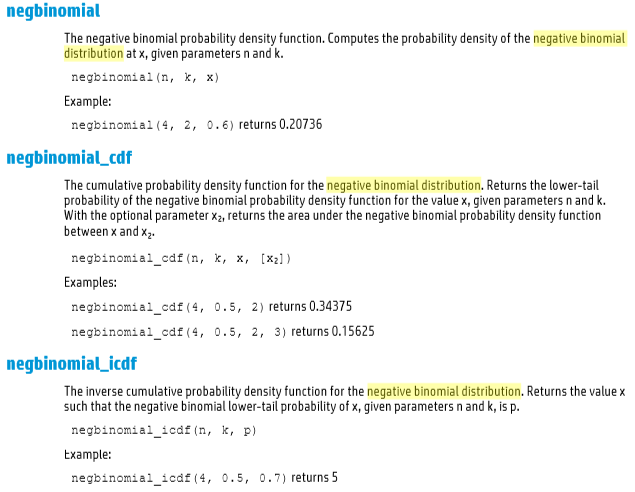cancel
Showing results for
Search instead for
Did you mean:It has been a while since anyone has replied. Simply ask a new question if you would like to start the discussion again.New member
1 0 0 0
Message 1 of 3
916
Flag Post

Solved!

# info about negative binomialHP Recommended
HP Prime Graphing Calculator
Chrome OS

hello i have some issues about the negative binomial distribution

the help in the calculator doesnt specify what are the parameters that i need in the negbinomial function.

it says that there are a n,k, and x.

i want to know what values the function negbinomial (n,k,x) need to reproduce the answer thanks

Tags (2)
2 REPLIES 2Level 9
3,551 3,490 180 541
Message 2 of 3
Flag PostHP Recommended

Hi!, @jose_cardenas:

You can see, from User Guide, in page 445 of Catlg Menu ... https://support.hp.com/us-en/product/hp-prime-graphing-calculator/5367459/manualshttps://www.wolframalpha.com/input/?i=negative+binomial+distribution&rawformassumption=%7B%22F%22,+%...If it has served you, to solve, your problem, you can grant, as Solved. In this way, it can serve other users. Also, you can grant Kudos, if you want to thank, the help provided.

Kind Regards !.
Have a nice day !.
@Maké (Technical Advisor Premium - HP Program Top Contributor).
Provost in HP Spanish Public Forum ... https://h30467.www3.hp.com/Level 6
305 297 32 76
Message 3 of 3
Flag PostHP Recommended

@jose_cardenas wrote:

hello i have some issues about the negative binomial distribution

the help in the calculator doesnt specify what are the parameters that i need in the negbinomial function.

it says that there are a n,k, and x.

i want to know what values the function negbinomial (n,k,x) need to reproduce the answer thanks

The Help information is insufficient here.

n = the number of successes, including the last one.

k = the number of failures

x = probability that an individual trial is a succes.

So the total number of trials is n+k.

Negbinomial (n,k,x) gives the probability of observing the nth success at the (n+k)th trial.

Tags (2)
† The opinions expressed above are the personal opinions of the authors, not of HP. By using this site, you accept the Terms of Use and Rules of Participation